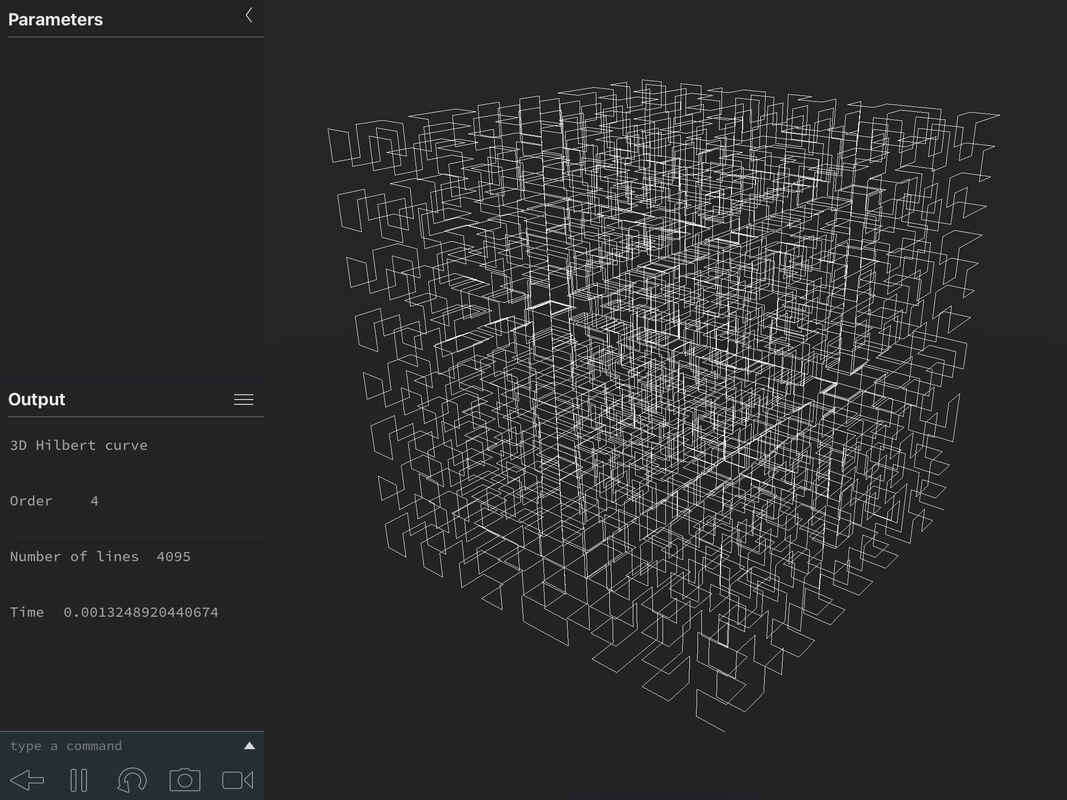I made a 2D Hilbert Curve grapher.

Here is the code

Code:
```ClrDraw:AxesOff:FnOff 0->Xmin:320->Xmax:0->Ymin:240->Ymax BackgroundOn Black Input "ORDER:",O .5^OAns->Q .5(Ans+Ans[i]->Z Ans->R {0,[i],1+[i],1->L2 For(I,1,4^O-1    I->M    remainder(Ans,4->L    L2(1+Ans->V    For(J,1,O-1       int(M.25->M       remainder(Ans,4->L       If not(Ans       Then          [i]conj(V->V       Else          2^J          If L=1          Then             V+Ans[i]->V          Else             If L=2             Then                V+Ans+Ans[i]->V             Else                [i]Ans-[i]+2Ans-1-[i]conj(V->V             End          End       End    End    QAns+R    Line(real(Z),imag(Z),real(Ans),imag(Ans),1,White,1    Ans->Z End```

EDIT: Incorporated some of the optimizations from other users.
(The inner loops are optimized for speed rather than size since they can be called thousands of times)

Here are some curvesNote: It graphs the curves "upside down" because the Y axis is inverted from a normal cartesian plane (Y=0 is the top and Y=240 is the bottom)
This could be easily fixed by adding "240-" before the Y coordinates in the Line() command.

This post gives off Barnsley's Fern vibesWOW! Great job!.
I'm just trying to understand the code to know for myself how to make such programs.

edit: 2D Curve? What would a 3D Hilbert curve look like? (not necessarily on a calculator, but in general)
DAVID-19 wrote:
WOW! Great job!.
I'm just trying to understand the code to know for myself how to make such programs.

edit: 2D Curve? What would a 3D Hilbert curve look like? (not necessarily on a calculator, but in general)

I took inspiration from the iterative algorithm described in this blog post.
A 3D Hilbert Curve is really the same thing with an extra dimension, it fills volume instead of area like this.
2D space filled with an infinite curve.
3D space filled with an infinte curve too.

I love it to fill (almost) every pixel on the graph screen with this curve.
It looks better than filling the screen with vertical lines of pixels.
Optimized for size:

Code:
```ClrDraw:AxesOff:FnOff 0->Xmin:320->Xmax:0->Ymin:240->Ymax BackgroundOn Black Input "ORDER:",O 240/(2^O->Q .5(Q+Q[i]->Z Ans->R For(I,1,4^O-1    I->M    {0,[i],1+[i],1    Ans(1+remainder(M,4->V    For(J,1,O-1       int(M/4->M       remainder(Ans,4->L       2^J->B       V+Ans[i]       If L=2       Ans+B       If L=3       [i]B-[i]+2B-1-[i]conj(V       If not(L       [i]conj(V       Ans->V    End    QAns+R    Line(real(Z),imag(Z),real(Ans),imag(Ans),1,White,1    Ans->Z End```

It runs significantly slower thoCode:
```ClrDraw:AxesOff:FnOff 0->Xmin:320->Xmax:0->Ymin:240->Ymax BackgroundOn Black Input "ORDER:",O .5^OAns->Q .5(Q+Q[i]->Z Ans->R For(I,1,4^O-1    I->M    {0,[i],1+[i],1    Ans(1+remainder(M,4->V    For(J,1,O-1       int(M/4->M       remainder(Ans,4->L       2^J->B       V+Ans[i]       If L=2       Ans+B       If L=3       [i]B-[i]+2B-1-[i]conj(V       If not(L       [i]conj(V       Ans->V    End    QAns+R    Line(real(Z),imag(Z),real(Ans),imag(Ans),1,White,1    Ans->Z End```

Made a small change to the setup that removes a couple of bytes from PT_'s code.
mr womp womp; Enjoyed the Hilbert code. Keyed it on my calc just to try it out. I wanted to convert it to lua code to run on my iPad, but I couldnt figure out the code that used the imaginary commands. I gave up after awhile and decided to just look on the internet to find some lua code. Couldnt find anything that was usable, but I did find some pseudo code so I went from that and wrote my own. When I ran the order 5 on my calc, it took 1 min 55 sec, on my iPad it took .014 sec. Just in case anyone is interested, heres the order 8 image. It took around .14 seconds and drew 65,535 lines.Heres an example of a 3D Hilbert curve that was asked about above. I found some code that I converted to Lua for my iPad. Looking at this picture doesnt give a good representation of the curve. On my iPad, I can zoom in or out, or rotate the curve in any direction. As you rotate the curve, you can see all the twists and turns easily. In the picture, everything is just jumbled together.Version using Stat Plots that nobody asked for:

Code:
```ClrDraw:AxesOff:FnOff Plot1(xyLine,L1,L2,dot,WHITE {0,[i],1+[i],1->L3 0->Xmin:320->Xmax 0->Ymin:240->Ymax BackgroundOn BLACK Input "ORDER:",O Ans/2^0->Q .5AnsL3(3->R:{Ans->L1 For(I,1,4^O-1:I->M     L3(1+remainder(Ans,4->V     For(J,1,I-1         2^J->B:int(M.25->M         remainder(Ans,4->L         If Ans=3 or not(Ans:Then             [i]conj(V             If L=3:2B+B[i]-Ans-L3(3         Else             V+B[i]             If L=2:Ans+B         End:Ans->V      End      QAns+R->L1(1+dim(L1 End imag(L1->L2:real(L1->L1 DispGraph ```

Obviously little change to speed and size, and nothing interesting happens while the list is being calculated, but there is something satisfying about the curve rapidly being drawn once it's done.

Register to Join the Conversation
Have your own thoughts to add to this or any other topic? Want to ask a question, offer a suggestion, share your own programs and projects, upload a file to the file archives, get help with calculator and computer programming, or simply chat with like-minded coders and tech and calculator enthusiasts via the site-wide AJAX SAX widget? Registration for a free Cemetech account only takes a minute.

»
» All times are GMT - 5 Hours

You cannot post new topics in this forum
You cannot reply to topics in this forum
You cannot edit your posts in this forum
You cannot delete your posts in this forum
You cannot vote in polls in this forum

Advertisement